Scientific

# 2D Correlation Analysis

In 2005, I developed the 2D gel correlation technique because it was the only viable method to analyze the data we received at that time. The company I worked for tried to patent this method (which was a silly thing to do anyway, its software) and received feedback from the UK patent office that the method was already 'invented' in 1975. Of course, since the 70's improvements in computer processing power makes that the method can now produce larger volume results. If you are interested in a demonstration dataset then please have a look here

# Overview

The method correlates the content of multiple 2D gels with some external parameter (survival rate for instance) and will report the correlation using a hue color scheme. There is a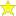movie available that summarizes the following steps. In the examples below, nothing of the biological information is correct. The examples give an overview of how the method work and don't make any biological sense. Even the reference to the 'age' parameter is wrong.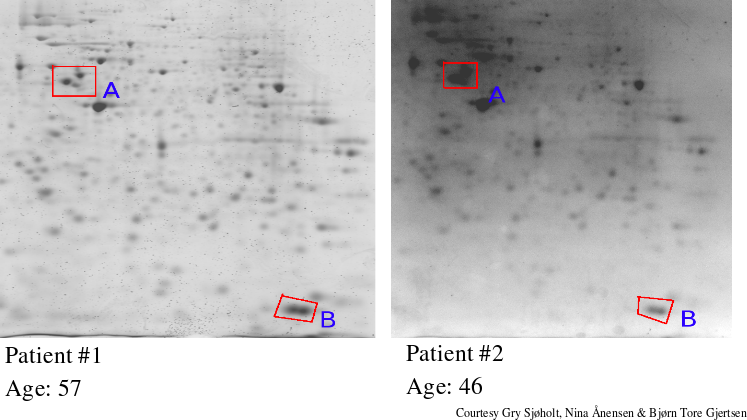First one starts with a large collection of 2D gels (for instance of AML/ALL patients). Each of these gels is associated with an extra parameter, such as survival rate, age, disease type and so on. The question one now asks is whether there is a relation between spots on the 2D gels and that specific parameter. E.g: does specific spots correlate towards the age ?

## Step 1: Align all images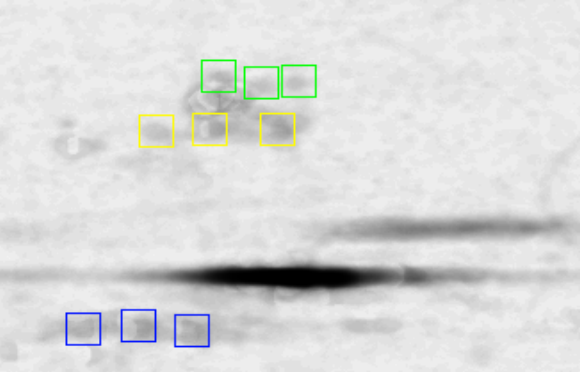The first step in the process concerns itself with aligning all the images such that each pixel on each aligned image reflects the same pI (horizontally) and protein mass (vertically). This step might require rotation, zooming and translation of each individual image. Important here is to realize that cumulative superposition schemes do not work properly. The image above shows the superposition of 128 aligned images.

## Step 2: Normalize all images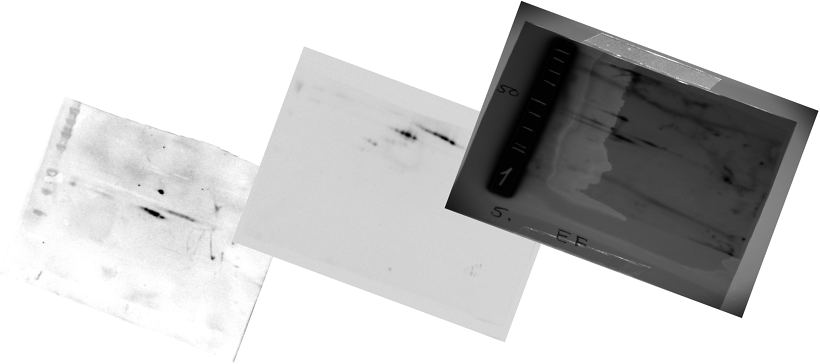Once the images are aligned, we need to make sure that we can compare the data between the different images. Often this might require contrast and brightness alterations. E.g: In the images above we clearly see that the background is different on the three images. Normalization will remove these differences.

## Step 3: Correlate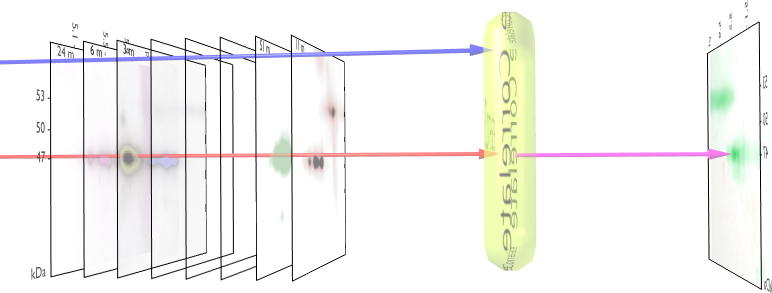The correlation image can then be computed using for instance the Spearman Rank order correlation. This is done on an individual pixel basis and leads to images such as the following: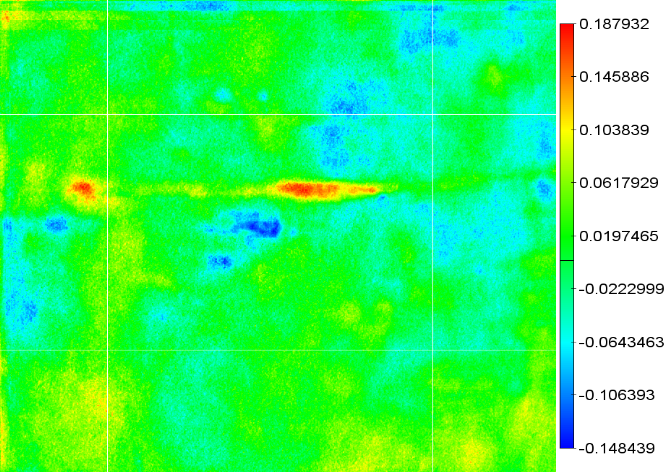The problem with the above image is that it shows some useless information. Areas on the gel where we have a correlation but where the gel content does not alter sufficiently are not useful. Neither are areas where the probability that that specific correlation might occur by chance. To mask these areas we create two new images. One for the variability on the gel and one for the correlation significance. See figures below: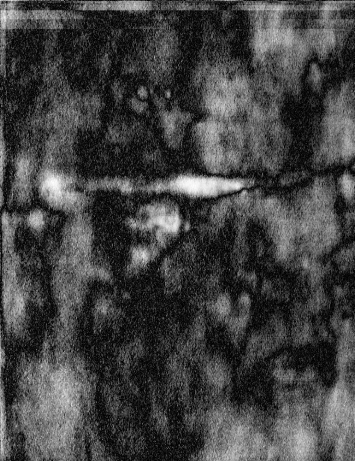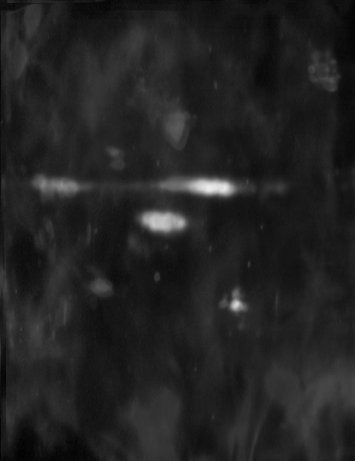The significance mask The variance mask
When these two masks are combined into the correlation image we get the much more understandable correlation map: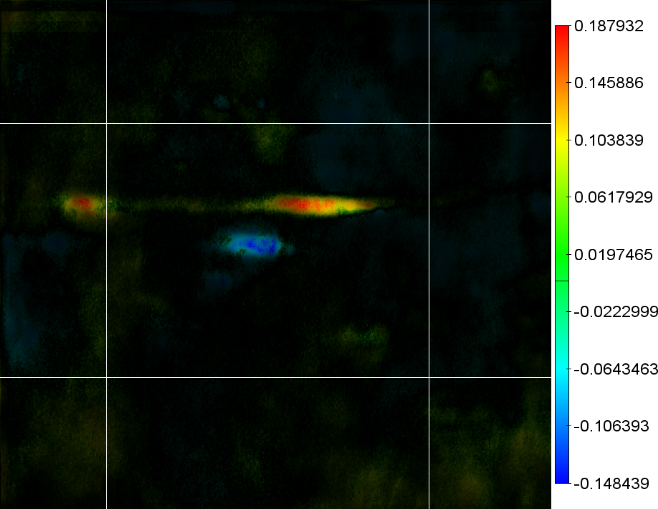In this image, the areas where the image is red reflect the areas on the gels where we will tend to find protein for elderly patients. Where the image is blue we will find that older patients have less protein expression.

## Step 5: Creating the 3D volumetric map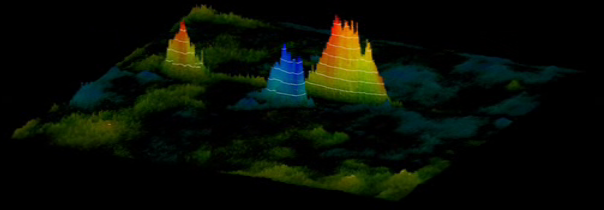To have a better understanding of which spots correlate with the external parameter it is useful to map this 2D gel in 3 dimensions. The 3th dimension is then used to reflect the correlation strength. Such volumetric maps can even be rotated:Rotating the 3D volumetric view of the 2D correlation map

# Ordering

If you are interested in this analysis feel free to contact werner@yellowcouch.org with the following tentative information: checklist
- http://analysis.yellowcouch.org/